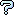All about flooble | fun stuff | Get a free chatterbox | Free JavaScript | Avatarsperplexus dot infoMinimum area of a triangle (Posted on 2008-07-30)Find the minimum area of a triangle whose sides and altitudes are six different integers.

 See The Solution Submitted by pcbouhid Rating: 2.0000 (2 votes)Comments: ( Back to comment list | You must be logged in to post comments.)re: Computer Solution| Comment 2 of 10 |(In reply to Computer Solution by Bractals)

Am I missing something?

By definition of a triangle's altitude, h_a would be perpendicular to side a.  This would mean, in accordance to Pythagoras, that
a1 = SQRT((75)2 - (60))2) and a2 = SQRT((100))2 - (60))2), where a = a1 + a2.  Thus a1 and a2 equals 45 and 80, respectively, which means side a should equal 125, not 35.

 Posted by Dej Mar on 2008-07-30 15:04:45Please log in:

 Search: Search body:
Forums (0)